# GMAT Math : DSQ: Calculating the equation of a parallel line

## Example Questions

### Example Question #1 : Dsq: Calculating The Equation Of A Parallel Line

Data Sufficiency Question

What is the slope of a line that passes through the point (2,3)?

1. It passes through the origin

2. It does not intersect with the line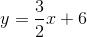both statements taken together are sufficient to answer the question, but neither statement alone is sufficient

statement 1 alone is sufficient, but statement 2 alone is not sufficient to answer the question

statements 1 and 2 together are not sufficient, and additional data is needed to answer the question

statement 2 alone is sufficient, but statement 1 alone is not sufficient to answer the question

each statement alone is sufficient

each statement alone is sufficient

Explanation:

In order to calculate the equation of a line that passes through a point, we need one of two pieces of information. If we know another point, we can calculate the slope and solve for the-intercept, giving us the equation of the line. Alternatively, if we know the slope (which we can conclude from the parallel line in statement 2) we can calculate the-intercept and determine the equation of the line.

### Example Question #2 : Dsq: Calculating The Equation Of A Parallel Line

Find the equation of the line parallel to the following line: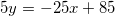I) The new line passes through the point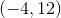.

II) The new line has a-intercept of.

Statement I is sufficient to answer the question, but statement II is not sufficient to answer the question.

Both statements are needed to answer the question.

Statement II is sufficient to answer the question, but statement I is not sufficient to answer the question.

Either statement is sufficient to answer the question.

Either statement is sufficient to answer the question.

Explanation:

To find the equation of a parallel line, we need the slope and the y-intercept.

Parallel lines have the same slope, so we have that.

I and II each give us a point on the graph, so we could find the equation of the line through either of them.

### Example Question #3 : Dsq: Calculating The Equation Of A Parallel Line

Find the equation of the line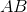.

1. The slope of lineis.
2. Linegoes through point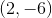.

Statement 2 alone is sufficient, but statement 1 alone is not sufficient to answer the question.

Statement 1 alone is sufficient, but statement 2 alone is not sufficient to answer the question.

Statements 1 and 2 are not sufficient, and additional data is needed to answer the question.

Each statement alone is sufficient to answer the question.

Both statements taken together are sufficient to answer the question, but neither statement alone is sufficient.

Both statements taken together are sufficient to answer the question, but neither statement alone is sufficient.

Explanation:

Statement 1: We're given the slope line AB, because we are ask for the equation of the line we need more than just the slope of the line. Therefore, this information alone is not sufficient to write an actual equation.

Statement 2: Using the information from statement 1 and the points provided in this statement, we can answer the question.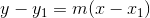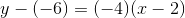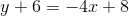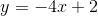### Example Question #4 : Dsq: Calculating The Equation Of A Parallel Line

Given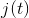, find the equation of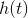.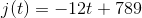I)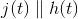II)passes through the point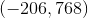Both statements are needed to answer the question.

Statement II is sufficient to answer the question, but Statement I is not sufficient to answer the question.

Either statement is sufficient to answer the question.

Statement I is sufficient to answer the question, but Statement II is not sufficient to answer the question.

Both statements are needed to answer the question.

Explanation:

We are asked to find the equation of a line related to another line.

Statement I tells us the two lines are parallel. This means they have the same slope

Statement II gives us a point on our desired line. We can use this to find the line's y-intercept, which will then allow us to write its equation.

Plug all of the given info into slope-intercept form and solve for b, the line's y-intercept: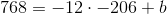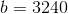So our equation is: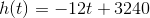Tired of practice problems?

Try live online GMAT prep today.